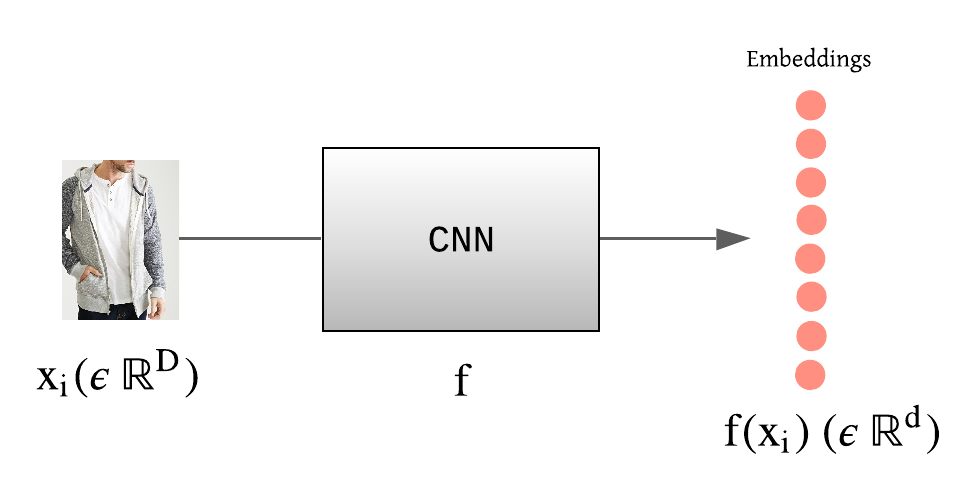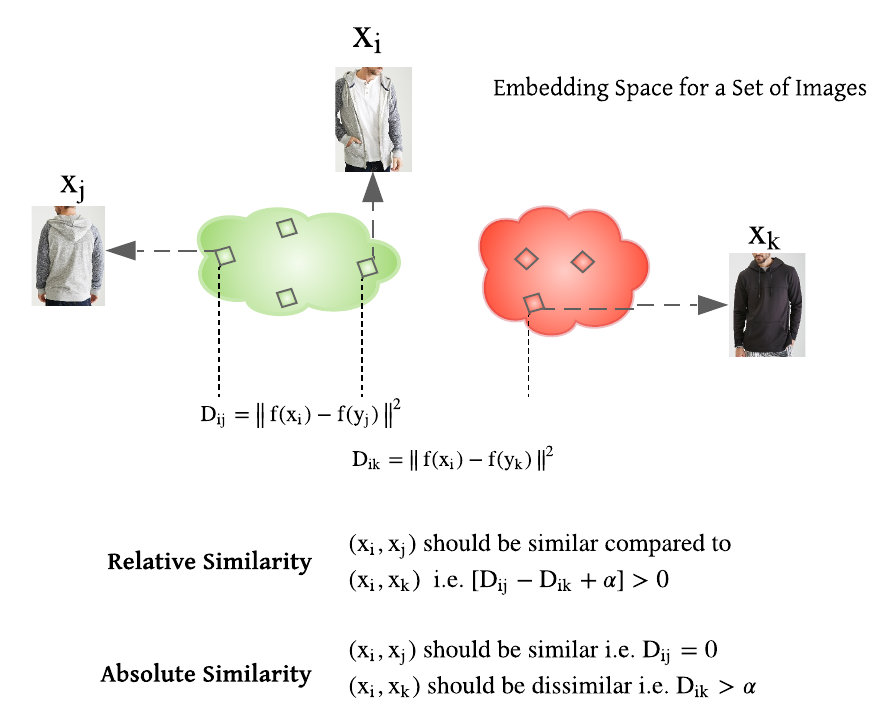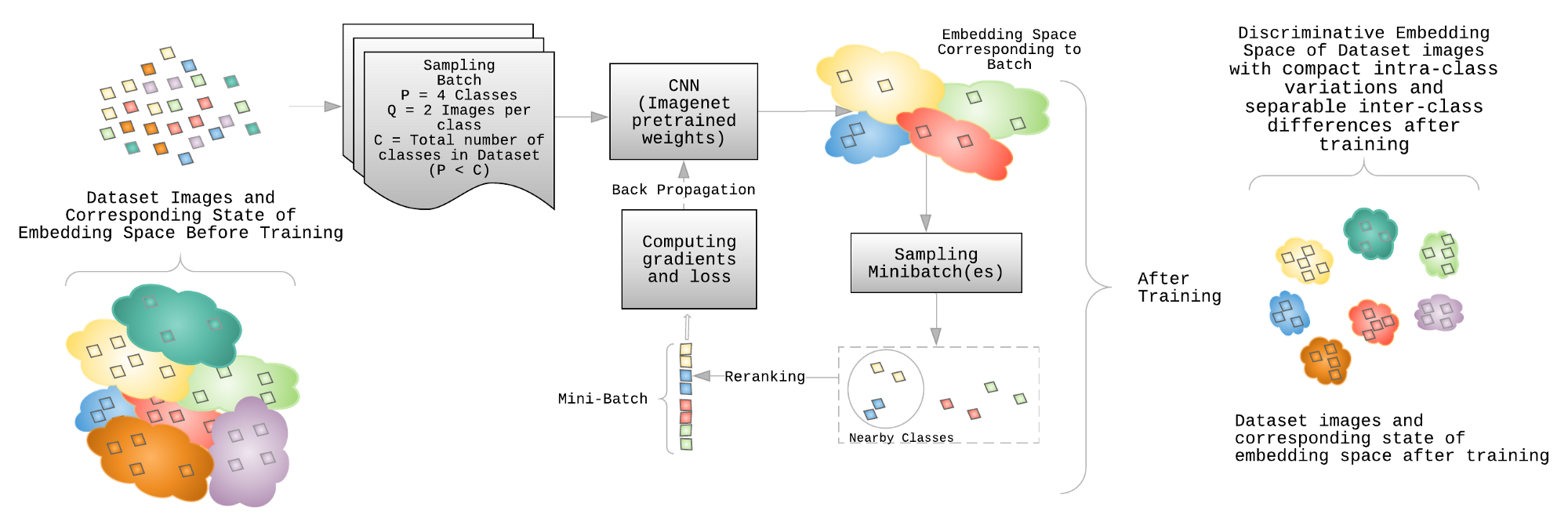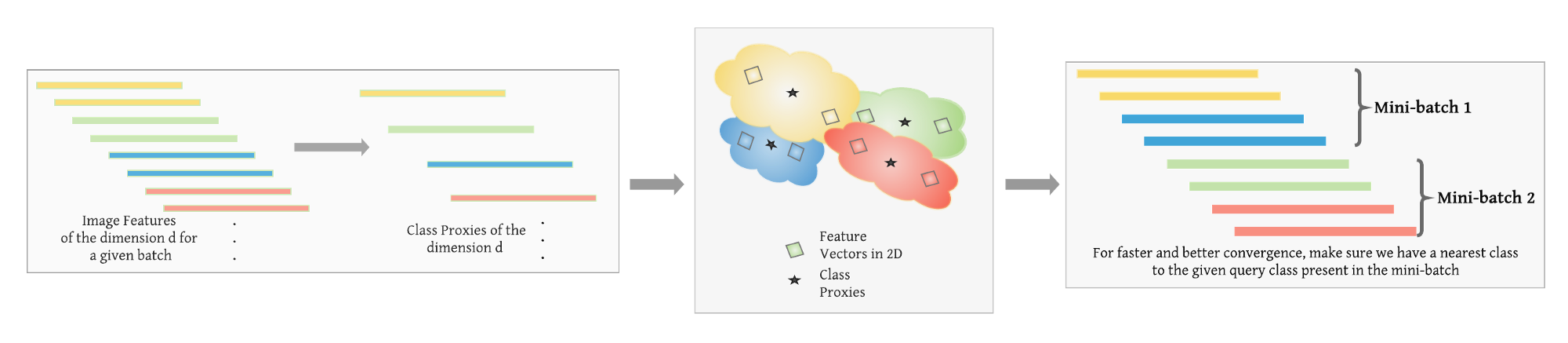- 增长的物体类别数，同时会增加 CNN 网络倒数第二层的权重参数量. 由于其不断增长的模型大小，导致其很难在设备上部署.

 - 当图片类里仅有少量几张图片时，分类模型很难较好的收敛，使其很难在不同光照变化、尺度、背景复杂、缺失遮挡等情况时取得较好的效果.

 - 实际工程中，往往需要视觉搜索系统能够适用于多种产品生态系统，如数据集中没有满足的产品、随着地理位置或季节趋势发生变化等. 这些情况使其很难采用周期性或实时性的方式进行模型的训练或微调.

## 1. 深度度量学习

DML 提出训练基于 CNN 的非线性特征提取模块(或编码器，encoder)，使得提取的具有语义相似性的图像特征(也叫嵌入，embeddings) 是相互临近的，而采用适当的距离度量(如 Euclidean 或 Cosine 距离)使得不相似的图像特征是彼此远离的.## 2. 问题阐述$$D_{ij} = ||f(x_i) - f(x_j)||^2$$- 相似性约束

$R = \lbrace (x_i, x_j, x_k): x_i 和 x_j 比和 x_k 更相似 \rbrace$

$D_{ij} - D_{ik} + \alpha > 0$ 量化了 anchor-positive 图像对和 anchor-negative 图像对之间的相似性.

 - 绝对性约束

$S = \lbrace (x_i, x_j): x_i 和 x_j 相似 \rbrace$.

$D = \lbrace (x_i, x_k): x_i 和 x_k 不相似 \rbrace$.

$D_{ij}$ 和 $D_{ik}$ 分别量化了正样本图像对和负样本图像对的相似性.

## 3. 模型训练### 3.1. 训练流程

 - Batch 采样：

Batchsize B，类别数目 P，每个类别的图片数量 Q.

 - 输入：

 - 特征提取：

 - 采样：

 - 损失计算和训练

### 3.2. 损失函数

CNN 分类模型，一般是采用 Softmax Cross-Entropy (CE) Loss 损失函数.

 - Softmax Cross-Entropy (CE) Loss 可以看作是 max 操作子的 soft 版本. 如果采用常数隐私 $s$ 进行缩放，Logit 向量或类别概率并不影响给定图片的类目分配. 因此，可良好分离的特征具有更大的幅度，且能够促进类别的可分离性. 总之，这将导致特征分布呈放射状，如下图所示. 且，如果使用具有区分性的特征学习算法，如 KNN 分类器，对特征进行分类，则拥有不仅可分离而且可区分性的嵌入空间是至关重要的. 度量学习的损失函数旨在学习具有可区分性的特征空间.- CE Loss 并未直接利用 mini-batch 图像的结构性关系，因为每张图片(随机选取)是分别进行损失函数计算. 通过引入一种能够解决图像之间的语义差异性的惩罚，可以区分图像对或图像三元组的训练范式，以有效的检查图像之间的关系.

Lifted Structure Loss 充分利用 mini-batch 的数据，提升 mini-batch 的SGD 训练. 另一方面，三元组损失或对比损失分别利用图像三元组和图像对进行损失计算, Lifted Structure loss 提出采用成对距离度量 (O(m^2))，利用mini-batch 内所有的图像对(O(m)). 此外，相比于三元损失和对比损失，负样本仅是关于 一张 anchor 图像进行定义的，Lifted Structure Loss 同时利用给定图像对中的 anchor 样本和正样本，来从 mini-batch 内的图像中找寻其负样本.

Lifted Structure Loss 公式如：

$$L_{i,j} = log(\sum_{(i, k) \in N} e^{(\alpha - D_{i,k})} + \sum_{(i, l) \in N}e^{(\alpha - D_{j, l})} ) + D_{i,j}$$

$$L = \frac{1}{2|P|} \sum_{(i, j) \in P} max(0, L_{i,j})^2$$

$D_{i,k}$ 和 $D_{j,l}$ 表示 mini-batch 内 anchor 和正样本与剩余负样本图像之间的距离.

$\alpha$ 表示距离间隔(margin).

$P$ 和 $N$ 分别表示 mini-batch 内所有的正样本对和负样本对.

### 3.3. 采样

DML 训练机制中，分别使用图像对或图像三元组的绝对相似性或相对相似性，在将图像输入到 CNN 时，需要有意义地采样图像 batches.- 对于给定参数状态 $\theta_t$，为了得到有意义的嵌入空间表示， 采样得到包含几百个类别的大的图像 batch(上面训练流程里是 P ). 对于每个图像对的损失函数，必须在给定 batch 中为每个给定类别分配 2 个图像样本. 例如，Stanford Online Products 数据集中，最少随机提取 2 张图片.

 - 对给给定 CNN 模型的给定参数状态 $\theta_t$，对  中采样的每张图片进行特征提取. 采用提取得到的图像特征得到类别表示向量或类别 proxies(嵌入向量).

 - 对于从  中采样的 P 个类别中每个随机选择的类别，采样其最临近的类别，并重排对应的图片. 这一步还可以采用基于 margin 的类别选择方法进行，如果一个类别违反距离 margin，则被选择作为给定 anchor 类别的最近邻类别.

 - 根据计算容量，构建一个或多个 mini-batch 的特征向量，以计算损失函数和梯度.

## 4. 模型评估和推断DML 特征评估的标准度量方式有：F1，NMI(Normalized Multual Information). 对于检索任务，recall@k 是常用的基准评测方式.

 - Recall@K

Recall@K 表示对测试集中所有结果的召回数量.

 - F1

F1 的计算为：F1=2PR/(P+R)

 - NMI

$$NMI = \frac{I(\Omega; C)}{2(H(\Omega) + H(C))}$$

 - Acc@K

Acc@K 对测试集中每张待查询图像的结果求均值.

## 5. 总结

 - 模型大小(model size) 不增加，因为模型训练的嵌入维度往往保持不变.

 - 由于目标是学习可区分性，而不是识别类别，因此可以对于每个类别仅有少量图片时，采用不同的采样策略进行训练和推断识别，即使是从未见过的类别.

 - 以周期性或在线方式微调到新的产品类别，都与梯度传播有关.

## 参考

1. Wang, X., Hua, Y., Kodirov, E., Hu, G., Garnier, R., & Robertson, N. M. (2019). Ranked list loss for deep metric learning. In Proceedings of the IEEE Conference on Computer Vision and Pattern Recognition (pp. 5207–5216).
2. Movshovitz-Attias, Y., Toshev, A., Leung, T. K., Ioffe, S., & Singh, S. (2017). No fuss distance metric learning using proxies. In Proceedings of the IEEE International Conference on Computer Vision (pp. 360–368).
3. Ranjan, R., Castillo, C. D., & Chellappa, R. (2017). L2-constrained softmax loss for discriminative face verification. arXiv preprint arXiv:1703.09507.
4. Wang, F., Xiang, X., Cheng, J., & Yuille, A. L. (2017, October). Normface: L2 hypersphere embedding for face verification. In Proceedings of the 25th ACM international conference on Multimedia (pp. 1041–1049).
5. Wu, C. Y., Manmatha, R., Smola, A. J., & Krahenbuhl, P. (2017). Sampling matters in deep embedding learning. In Proceedings of the IEEE International Conference on Computer Vision (pp. 2840–2848).
6. Wen, Y., Zhang, K., Li, Z., & Qiao, Y. (2016, October). A discriminative feature learning approach for deep face recognition. In European conference on computer vision (pp. 499–515). Springer, Cham.
7. Liu, Z., Luo, P., Qiu, S., Wang, X., & Tang, X. (2016). Deepfashion: Powering robust clothes recognition and retrieval with rich annotations. In Proceedings of the IEEE conference on computer vision and pattern recognition (pp. 1096–1104).
8. Oh Song, H., Xiang, Y., Jegelka, S., & Savarese, S. (2016). Deep metric learning via lifted structured feature embedding. In Proceedings of the IEEE conference on computer vision and pattern recognition (pp. 4004–4012).
9. Sohn, K. (2016). Improved deep metric learning with multi-class n-pair loss objective. In Advances in neural information processing systems (pp. 1857–1865).
10. Schroff, F., Kalenichenko, D., & Philbin, J. (2015). Facenet: A unified embedding for face recognition and clustering. In Proceedings of the IEEE conference on computer vision and pattern recognition (pp. 815–823).
11. Bellet, A., Habrard, A., & Sebban, M. (2013). A survey on metric learning for feature vectors and structured data. arXiv:1306.6709.
12. Hadsell, R., Chopra, S., & LeCun, Y. (2006, June). Dimensionality reduction by learning an invariant mapping. In 2006 IEEE Computer Society Conference on Computer Vision and Pattern Recognition (CVPR’06) (Vol. 2, pp. 1735–1742). IEEE. Chicago
Last modification：April 12th, 2021 at 04:25 pm